# Faculty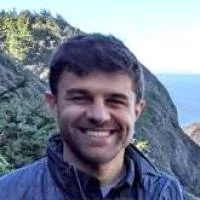### Christos Thrampoulidis

Research interests: High-dimensional signal processing, machine learning, optimization, statistics, mathematical data science### Danica Sutherland

Learning and testing on sets and distributions: two-sample tests, evaluating and training implicit generative models (e.g. GANs), density estimation, distribution regression.

Learning “deep kernels,” and representation learning more broadly; Statistical learning theory in general.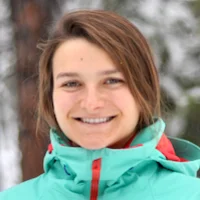### Elina Robeva

My research lies at the intersection of mathematical statistics, machine learning, combinatorics, multilinear algebra, and applied algebraic geometry. Most recently I have been working on the theory of linear causal models, structured tensor decompositions, shape-constrained density estimation, and super-resolution imaging.### Geoff Schiebinger

Research interests: The interplay between theory and experiment in natural science. New measurement technologies like single-cell RNA sequencing are bringing ‘big data’ to biology. My group develops mathematical tools for analyzing time-courses of high-dimensional gene expression data, leveraging tools from optimal transport, at the intersection of probability, statistics, and optimization.### Lele Wang

My current research focuses on the information-theoretic approach to data science and machine learning. Several recent works in this direction include universal compression of graphical data, coding theoretical approach to distributed machine learning, statistical inference on graphs, ranking from pairwise comparison, etc.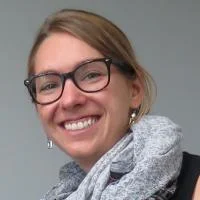### Lindsey Heagy

Machine learning, inverse problems### Mark Schmidt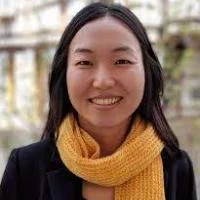### Mi Jung Park

Research Interests: Improving and developing methods in privacy-preserving machine learning.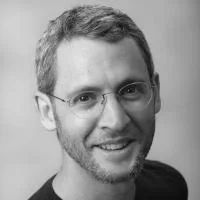### Michael Friedlander

Research interests: mathematical optimization, mathematics of information, high-dimensional data analysis, numerical methods.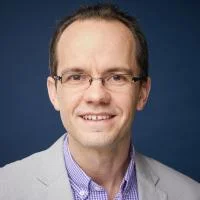### Nick Harvey

Research interests: algorithm design, combinatorial optimization, application of algorithms in computer systems and machine learning.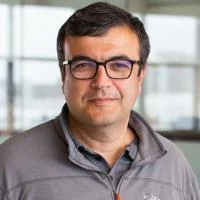### Ozgur Yilmaz

Applied and computational harmonic analysis, compressed sensing and related techniques, and mathematics of information and machine learning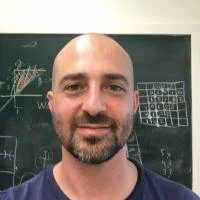### Yaniv Plan

Applied probability, compressive sensing, and matrix completion, and mathematical foundations of machine learning.

#### Steering Committee:

Nick Harvey (Chair)

Michael Friedlander

Yaniv Plan

#### Communications Committee:

Nick Harvey (Chair)

Michael Friedlander